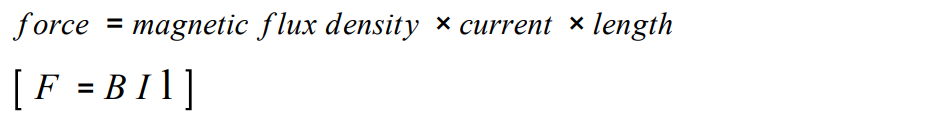# Questions on Fleming’s Left-hand rule for GCSE Physics #AQA #GCSE #Physics #revision #gcserevision

## Fleming’s Left Hand Rule and The Motor Effect

1. What happens when a conductor carrying a current is placed in a magnetic field?
2. What is this effect called?
3. In Fleming’s left-hand rule what do the thumb, first finger and second finger represent?
4. What are the factors that affect the size of the force on a conductor?
5. This equation is for a conductor at right angles to a magnetic field and carrying a current:6. What is the unit of force?
7. What is the unit of magnetic flux density?
8. What is the unit of current?
9. What is the unit of length?
10. Rearrange the equation so that force =, current =, length = and magnetic flux density =